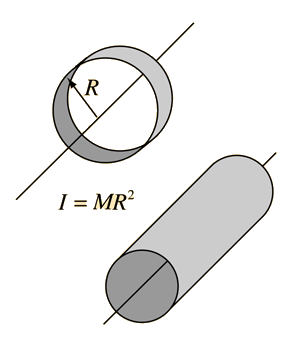# Moment of Inertia: HoopThe moment of inertia of a hoop or thin hollow cylinder of negligible thickness about its central axis is a straightforward extension of the moment of inertia of a point mass since all of the mass is at the same distance R from the central axis.

For mass M = kg
the moment of inertia is
I = kg m2
For a thin hoop about a diameter in the plane of the hoop, the application of the perpendicular axis theorem gives
I(thin hoop about diameter) = kg m2

### Show effect of hoop thickness

Index

Moment of inertia concepts

 HyperPhysics***** Mechanics R Nave
Go Back

# Thick Hoops and Hollow CylindersFor mass M = kg,